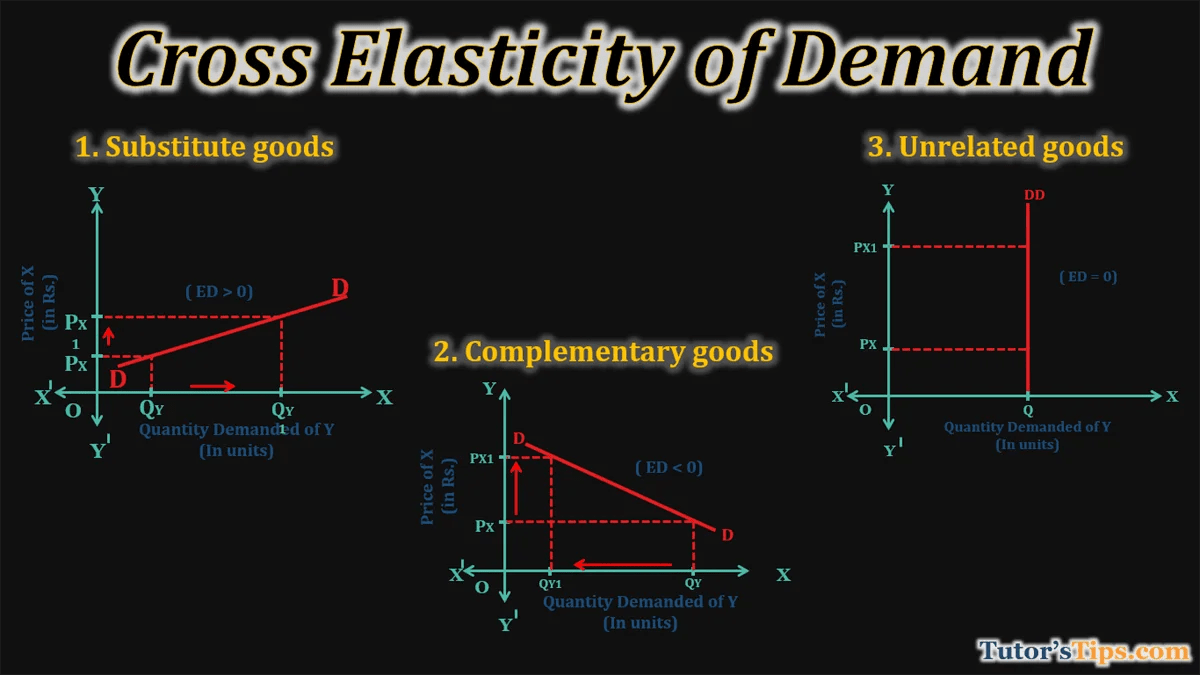# Cross elasticity of demand-Explanation with examplesCross elasticity of demand is referred to as the sensitivity of demand for one product to the price of another related product. It is the ratio of the percentage change in quantity demanded of good X and the percentage change in the price of good Y.

## Formula to calculate Cross Elasticity of Demand:

Cross elasticity = % change in quantity demanded of good X/ % change in the price of good Y% Δ quantity demanded of goods x = percentage change in quantity demanded
% Δ Price of goods y  = percentage change in Income of Consumer

## Types of cross elasticity of demand :

1. Substitute Goods
2. Complementary Goods
3. Unrelated Goods

### 1. Substitute goods:

When the cross elasticity of demand for good X relative to the price of good Y is positive, it means the goods X and Y are substitutes to each other. It implies that in response to an increase in the price of good Y, the quantity demanded of good X has increased as people start consuming product X as the price of good Y goes up.

For example, suppose a 10% increase in the price of tea results in an increase in demand for coffee by 15%. This shows that the goods are substitutes for each other.

### 2. Complementary goods:

When the cross elasticity of demand for good X relative to the price of good Y is negative, it means the goods are complementary to each other. It implies that in response to an increase in the price of good Y, the quantity demanded of good X has decreased due to the increase in the price of Y.

For example, suppose the 10% increase in the prices of Android phones results in a decline in the quantity demanded of Apps by 15%. This relation shows that the goods are complementary to each other.

### 3. Unrelated goods:

When the cross elasticity of demand for good X relative to the price of good Y is zero, it means goods are unrelated to each other. It implies that there is no relationship between these both goods.